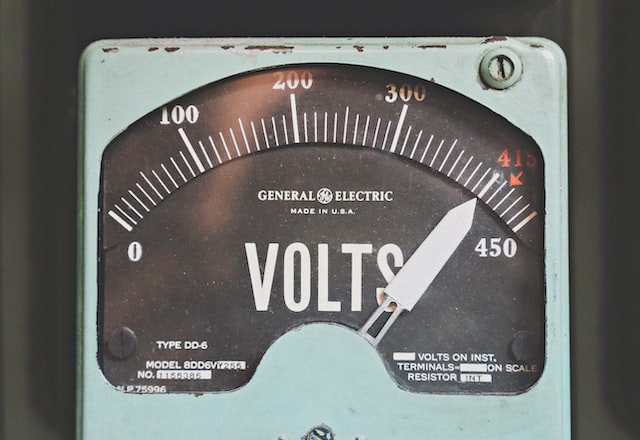Energy

## Part 1: What Is A KiloWatt, kWh and Other Energy Terms?

By

Team Ostrom

1.10.20223Electricity is a fundamental part of our daily lives, and it's important to have a basic understanding of the terms and units used to measure and describe it. Here is a summary of some common electricity terms:

1. Kilowatt (kW): A unit of power, equal to 1,000 watts. The rate at which energy is transferred or used, such as the power consumed by a device or the output of a power plant, is often measured in kilowatts.
2. Kilowatt hour (kWh): A unit of energy, equal to the energy consumed or produced by one kilowatt of power over a period of one hour. Electricity bills are often based on the number of kilowatt hours consumed by a home or business.
3. Ampere (A): A unit of electric current, equal to the flow of one coulomb of charge per second. The strength or intensity of an electric current is measured in amperes.
4. Volt (V): A unit of electric potential difference, equal to the work required to move a unit of charge from one point to another against an electric field. The electric potential difference between two points, or the force that drives the flow of electric charge, is measured in volts.
5. Watt (W): A unit of power, equal to one joule of energy per second. The rate at which energy is transferred or used, such as the power consumed by a device or the output of a power plant, is often measured in watts.
6. Lumen (lm): A unit of luminous flux, equal to the amount of light emitted in a unit of solid angle by a point source having a uniform intensity of one candle. The brightness or intensity of a light source is often measured in lumens.
7. Ohm (Ω): A unit of electrical resistance, equal to the resistance of a conductor in which a potential difference of one volt causes a current of one ampere. The resistance to the flow of electric charge through a conductor is measured in ohms.
8. Hertz (Hz): A unit of frequency, equal to one cycle per second. The number of cycles or vibrations per second of an alternating current or signal is measured in hertz.
9. Power factor (PF): A measure of the efficiency of an electrical system, equal to the ratio of the real power flowing to the load to the apparent power in the circuit. A power factor of 1 indicates that all the power being supplied to the load is being used, while a power factor less than 1 indicates that some of the power is being lost due to reactive power.
10. Direct current (DC): An electric current that flows in only one direction, as opposed to alternating current (AC), which periodically changes direction. Many electronic devices, such as computers and cellphones, operate on DC power.
11. Alternating current (AC): An electric current that periodically changes direction, as opposed to direct current (DC), which flows in only one direction. AC is the form of electricity commonly used in homes and businesses.

By understanding these basic electricity terms, you can better understand how electricity is generated, transmitted, and used in our daily lives.